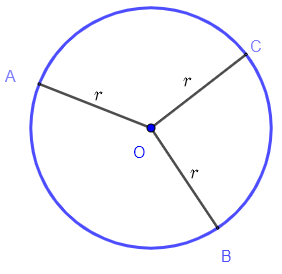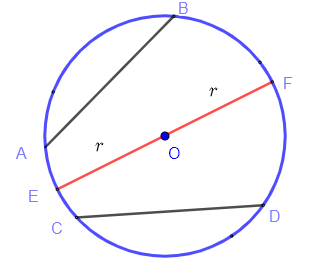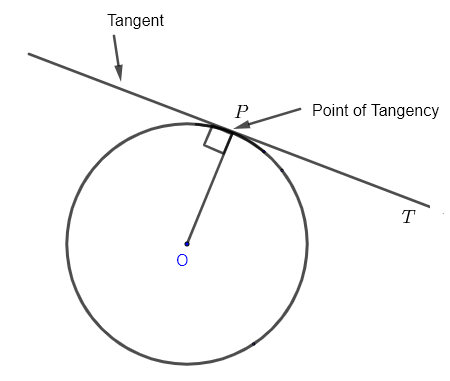# Parts of a Circle

A circle is a set of points in a plane that are equidistant from a point called the center of the cicle . In the figure below, $O$ is the center of the circle and points $A$, $B$ and $C$ are points on the circle at the same distance $r$ from the center $O$.The radius of a circle is the line segment from any point on the circle to the center of the circle. In the diagram above, $OA$, $OB$ and $OC$ are radii having the same length $r$.
The circumference of a circle is the perimeter of the circle or the total distance around the curved edge of a circle.
The formula of the circumference $C_i$ of a circle of radius $r$ is given by $C_i = 2 \pi r$
The formula of the area $A$ of the surface enclosed by a circle of radius $r$ is given by $A = \pi r^2$
A chord of a circle is any line segment joining two points that are on the circle. In the figure below, $AB$ , $EF$ and $CD$ are chords of the circle.
A diameter of a circle is a chord through the center of the circle. In the figure below, $EF$ is a diamter of the circle. The length of the diameter is equal to $2 r$, twice the length of the radius.An arc of a circle is a portion of a circle. In the figure below, two points $A$ and $B$ splits the circle inro two arcs with the small one called the minor arc and the large one called the major arc. The symbol $\overset{\LARGE\frown}{}$ is used above letters to represent an arc as follows: $\overset{\LARGE\frown}{AB}$.A central angle of a circle is an angle whose vertex is the center of the circle and whose legs are two radii. In the figure above, $\theta$ is a central angle.
The length $S$ of an arc corresponding to a central angle $\theta$ and in a circle of radius $r$ is given by $S = \theta r \quad \text{with} \; \theta \; \text {in radians}$
A
sector is a part of the circle enclosed by an arc and two radii. In the figure below, the blue surface is a sector.The area $A$ of a sector whose central angle $\theta$ and radius $r$ is given by $A = \dfrac{1}{2} \theta r^2 \quad \text{with} \; \theta \; \text {in radians}$

A straight line that touches a circle at one point is called tangent to a circle as shown in the figure below. The tangent $T$ touches the circle at the point of tangency $P$.